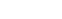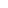# Linear Algebra and Differential Equations

Ordinary differential equations (ODEs) have solutions that depend on only one variable. Partial differential equations (PDEs) have solutions that depend on multiple variables.

Linear algebra is a language first, methodology second.

Keith Thompson

By order, we mean the highest order derivative that appear in the differential equation.

A solution to the above ODE is a functionthat reduces it to an identity given by:

For some appropriate domain points. You can also call this a solution, too:

A differential equation is called autonomous if the independent variable does not appear on both sides.

If you want to find thatthen you are solving an initial value problem. In order to determine that value for some ODE, we need to specify a condition called the initial value. Sometimes, it’s not always possible to solve a differential equation explicitly, so occasionally we view them as “direction fields” or sometimes called “slope fields.” Then there are also isoclines, which are super cool looking.

It would not be a linear algebra post without mention of one of the most used tools from that scientific field today: the matrix. No, not the one that Neo has to navigate. The one mathematicians use! It’s definition is super simple. It’s just a rectangular array of entries, arranged in rows and columns.

If you want an even more abstracted and more powerful version though, I suggest you read about tensors!

Posted

in

by